#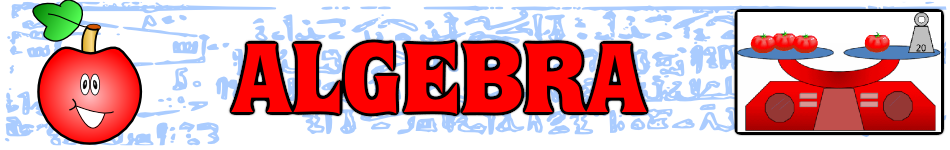There are 366 different Starters of The Day, many to choose from. You will find below some starters on the topic of Algebra. A lesson starter does not have to be on the same topic as the main part of the lesson or the topic of the previous lesson. It is often very useful to revise or explore other concepts by using a starter based on a totally different area of Mathematics.

Main Page

### Algebra Starters:Add up a sequence of consecutive numbers. Can you find a quick way to do it?This lesson starter requires pupils to find the missing numbers in this partly completed arithmagon puzzle.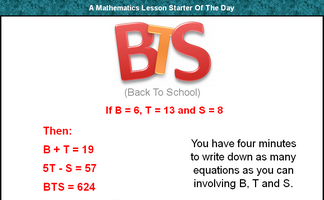You have four minutes to write down as many equations as you can involving B, T and S.Calculate the total cost of four cars from the information given.Work out the number of chin ups the characters do on the last day of the week give information about averages.Work out the total cost of five Christmas presents from the information given.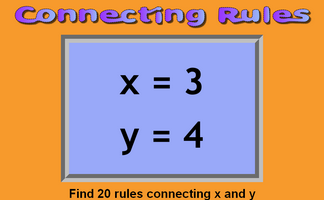Give 20 rules connecting x and y given their values.The height of this giraffe is three and a half metres plus half of its height. How tall is the giraffe?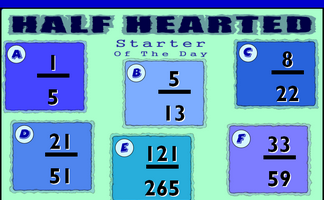Find the number which when added to the top (numerator) and bottom (denominator) of each fraction make it equivalent to one half.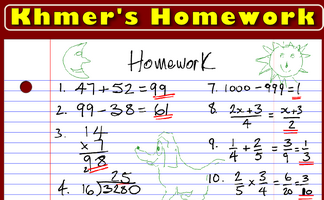Check a student's homework. If you find any of the answers are wrong write down a sentence or two explaining what he did wrong.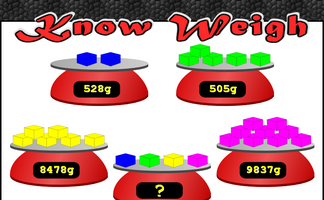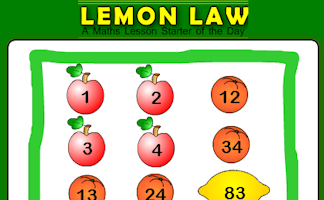Change the numbers on the apples so that the number on the lemon is the given total.This mathematics lesson starter invites pupils to interpret a three part algebraic inequality.A lamp and a bulb together cost 32 pounds. The lamp costs 30 pounds more than the bulb. How much does the bulb cost?Which algebraic expression is the odd one out?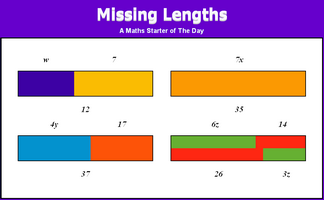Introduce linear equations by solving these problems about lengths.Work out why subtracting a two digit number from its reverse gives a multiple of nine.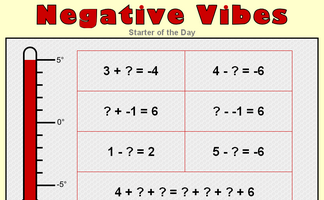Practise techniques for answering questions involving negative numbers.The sum and product are given, can you find the two numbers?You have four minutes to write down as many equations as you can involving the given letters.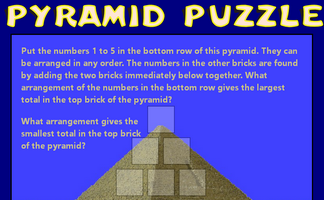Arrange numbers at the bottom of the pyramid which will give the largest total at the top.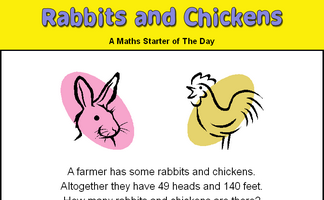There are some rabbits and chickens in a field. Calculate how many of each given the number of heads and feet.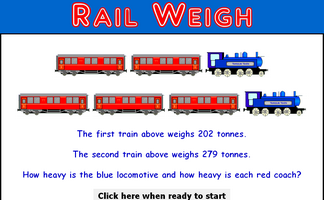Record the weights of the trains to work out the weight of a locomotive and a coach. A real situation which produces simultaneous equations.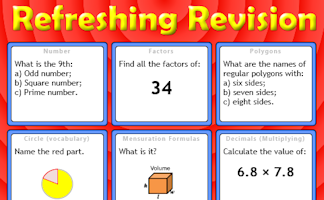It is called Refreshing Revision because every time you refresh the page you get different revision questions.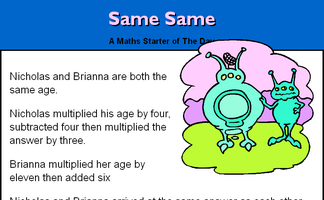A problem involving two people's ages which can be solved using algebra.Work out the number of clowns and horses given the number of heads and feet.A question which can be best answered by using algebra.A problem which can best be solved as a pair of simultaneous equations.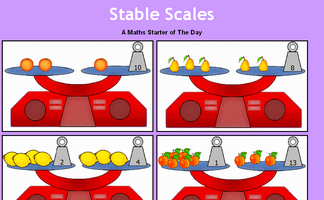Solve these balance puzzles by taking the same away from both sides. An introduction to linear equations.Each traffic sign stands for a number. Some of the sums of rows and columns are shown. What numbers might the signs stand for?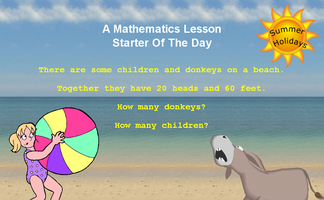How many children and how many donkeys are on the beach? You can work it out from the number of heads and the number of feet!A problem which can be answered by forming an algebraic equation then solving it.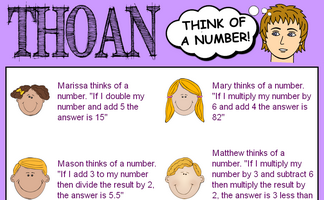THOAN stands for 'Think of a number' and there are four randomly generated THOAN puzzles to solve.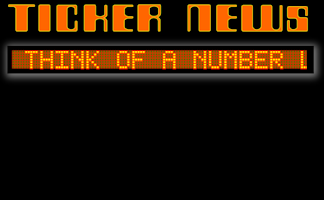A Think Of A Number problem presented as a news ticker.

## Exercises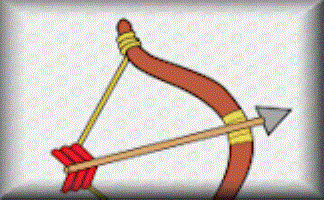#### Algebra In Action

Real life problems adapted from an old Mathematics textbook which can be solved using algebra.#### Algebra Pairs

The classic Pelmanism or pairs game requiring you to match equivalent expressions.#### Algebragons

Find the missing expressions in these partly completed algebraic arithmagon puzzles.#### Algebraic Fractions

A mixture of algebraic fraction calculations and simplifications.#### Algebraic Notation

Simplification using the normal conventions of algebra.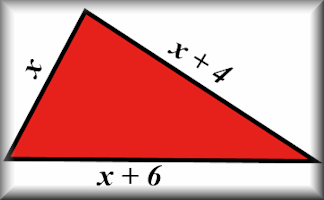#### Algebraic Perimeters

Questions about the perimeters and areas of polygons given as algebraic expressions.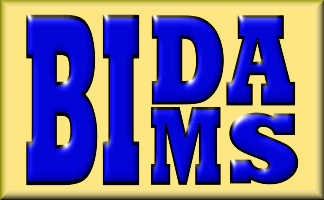#### BIDMAS

A self marking exercise testing the application of BIDMAS, an acronym describing the order of operations used when evaluating expressions.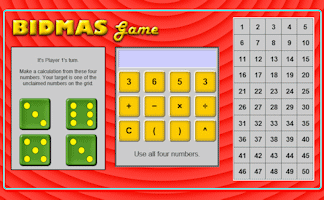#### BIDMAS Game

An online interactive game celebrating the order of mathematical operations.#### Binomial Theorem

Exercises in the process of expanding powers of binomial expressions and finding specific coefficients.#### Brackets

Expand algebraic expressions containing brackets and simplify the resulting expression in this self marking exercise.#### Calendar Maths Investigation

Investigate the connection between the numbers in a T shape drawn on this month's calendar.#### Changing The Subject

Rearrange a formula in order to find a new subject in this self marking exercise.#### Clouds

Can you work out which numbers are hidden behind the clouds in these calculations?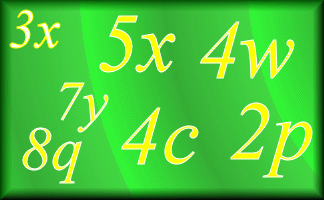#### Collecting Like Terms

Practise your algebraic simplification skills with this self marking exercise.#### Completing the Square

Practise this technique for use in solving quadratic equations and analysing graphs.#### Connecting Rules

If you are given the values of x and y which of these equations is correct?#### Crossing the River

Two men and two boys want to cross a river and they only have one canoe which will only hold one man or two boys.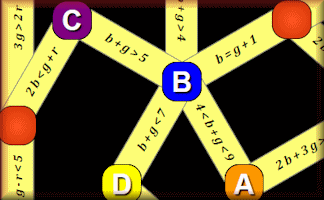#### DiceGebra

An online board game for two players evaluating algebraic equations and inequalities.#### eQuation Generator

An unlimited supply of linear equations just waiting to be solved. Project for the whole class to see then insert the working in your own style.#### Equations

A series of exercises, in increasing order of difficulty, requiring you to solve linear equations. The exercises are self marking.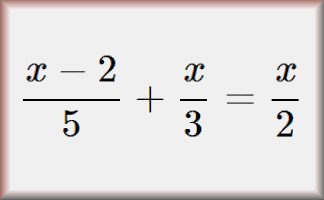#### Equations with Fractions

Practise solving linear equations that contain fractions in this multi-level exercise.#### Factorising

Practise the skills of algebraic factorisation in this structured online self marking exercise.#### Formulae to Remember

The traditional pairs or pelmanism game adapted to test recognition for formulae required to be memorised for GCSE exams.#### Function Builder

An interactive function machine for patterns, numbers and equations.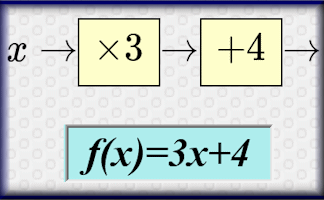#### Functions

An online exercise on function notation, inverse functions and composite functions.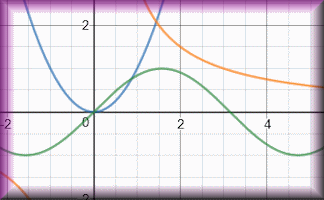#### Graph Equation Pairs

Match the equation with its graph. Includes quadratics, cubics, reciprocals, exponential and the sine function.#### How old was Diophantus?

An ancient riddle which can be answered by solving an equation containing fractions.#### Identity, Equation or Formula?

Arrange the given statements in groups to show whether they are identities, equations or formulae.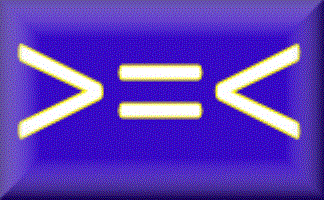#### Inequalities

Check that you know what inequality signs mean and how they are used to compare two quantities. Includes negative numbers, decimals, fractions and metric measures.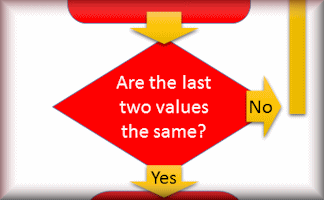#### Iteration

Find approximate solutions to equations numerically using iteration.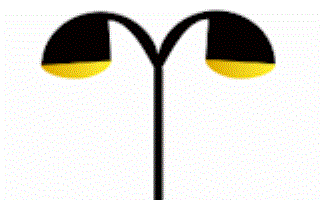#### Lamp Posts

What is the greatest number of lamp posts that would be needed for a strange village with only straight roads?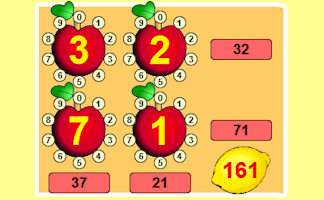#### Lemon Law

Change the numbers on the apples so that the number on the lemon is the given total.#### Linear Programming

A selection of linear programming questions with an interactive graph plotting tool.#### Matchstick Patterns

Create a formula to describe the nth term of a sequence by examining the structure of the diagrams.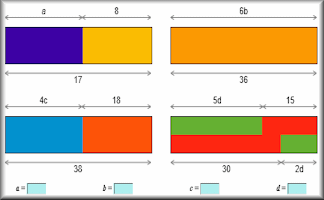#### Missing Lengths

Find the unknown lengths in the given diagrams and learn some algebra at the same time.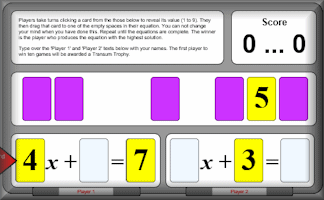#### Nevertheless

Players decide where to place the cards to make an equation with the largest possible solution.#### Old Equations

Solve these linear equations that appeared in a book called A Graduated Series of Exercises in Elementary Algebra by Rev George Farncomb Wright published in 1857.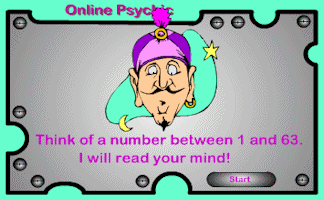#### Online Psychic

Let the psychic read the cards and magically reveal the number you have secretly chosen. What is the mathematics that makes this trick work?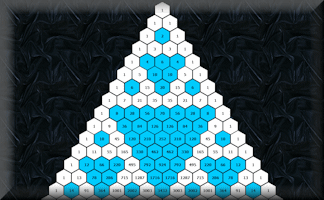#### Pascal's Triangle

Get to know this famous number pattern with some revealing learning activities#### Polynomial Division

Practise dividing one algebraic expression by another in this set of exercises.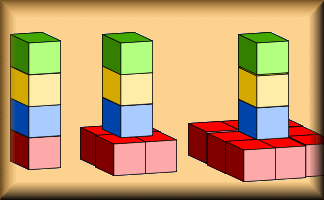Deduce expressions to calculate the nth term of quadratic and cubic sequences.Solve these quadratic equations algebraically in this seven-level, self-marking online exercise.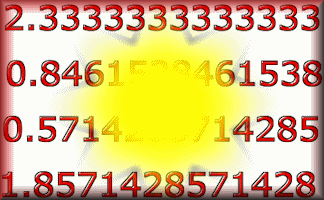#### Recurring Decimals

Change recurring decimals into their corresponding fractions and vica versa.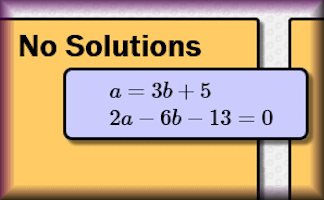#### Simultaneous Solutions

Arrange the given pairs of simultaneous equations in groups to show whether they have no solution, one solution or infinite solutions.#### Stable Scales

Ten balance puzzles to prepare you for solving equations.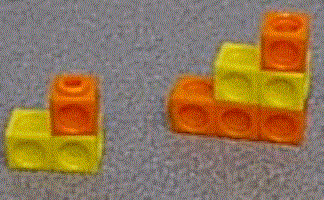#### Steps

Investigate the numbers associated with this growing sequence of steps made from Multilink cubes.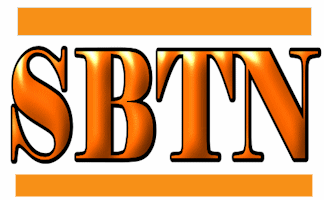#### Substitution

Substitute the given values into the algebraic expressions.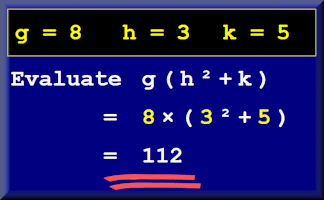#### Substitution Examples

A projectable set of animated examples to help prepare pupils to do the Substitution online exercise.#### Superfluous

Find a strategy to figure out the values of the letters used in these calculations.#### Think of a Number

Ten students think of a number then perform various operations on that number. You have to find what the original numbers were.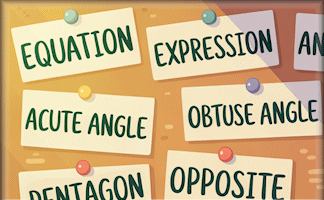#### Words and Concepts

Fill in the missing words to show an understanding of the vocabulary of equations, inequalities, terms and factors.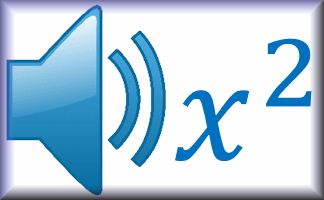#### Writing Expressions

Listen to the voice saying the algebraic expression then write it in its simplest form.

### Search

The activity you are looking for may have been classified in a different way from the way you were expecting. You can search the whole of Transum Maths by using the box below.

Have today's Starter of the Day as your default homepage. Copy the URL below then select
Tools > Internet Options (Internet Explorer) then paste the URL into the homepage field.

Set as your homepage (if you are using Internet Explorer)

Do you have any comments? It is always useful to receive feedback and helps make this free resource even more useful for those learning Mathematics anywhere in the world. Click here to enter your comments.For All: